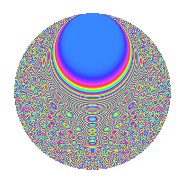# Properties

 Label 8013.2.aLevel 8013 Weight 2 Character orbit a Rep. character $$\chi_{8013}(1,\cdot)$$ Character field $$\Q$$ Dimension 445 Newforms 4 Sturm bound 1781 Trace bound 1

# Related objects

## Defining parameters

 Level: $$N$$ = $$8013 = 3 \cdot 2671$$ Weight: $$k$$ = $$2$$ Character orbit: $$[\chi]$$ = 8013.a (trivial) Character field: $$\Q$$ Newforms: $$4$$ Sturm bound: $$1781$$ Trace bound: $$1$$

## Dimensions

The following table gives the dimensions of various subspaces of $$M_{2}(\Gamma_0(8013))$$.

Total New Old
Modular forms 892 445 447
Cusp forms 889 445 444
Eisenstein series 3 0 3

The following table gives the dimensions of the cuspidal new subspaces with specified eigenvalues for the Atkin-Lehner operators and the Fricke involution.

$$3$$$$2671$$FrickeDim.
$$+$$$$+$$$$+$$$$116$$
$$+$$$$-$$$$-$$$$106$$
$$-$$$$+$$$$-$$$$129$$
$$-$$$$-$$$$+$$$$94$$
Plus space$$+$$$$210$$
Minus space$$-$$$$235$$

## Trace form

 $$445q$$ $$\mathstrut +\mathstrut q^{2}$$ $$\mathstrut +\mathstrut q^{3}$$ $$\mathstrut +\mathstrut 449q^{4}$$ $$\mathstrut -\mathstrut 2q^{5}$$ $$\mathstrut +\mathstrut 3q^{6}$$ $$\mathstrut +\mathstrut 8q^{7}$$ $$\mathstrut +\mathstrut 9q^{8}$$ $$\mathstrut +\mathstrut 445q^{9}$$ $$\mathstrut +\mathstrut O(q^{10})$$ $$445q$$ $$\mathstrut +\mathstrut q^{2}$$ $$\mathstrut +\mathstrut q^{3}$$ $$\mathstrut +\mathstrut 449q^{4}$$ $$\mathstrut -\mathstrut 2q^{5}$$ $$\mathstrut +\mathstrut 3q^{6}$$ $$\mathstrut +\mathstrut 8q^{7}$$ $$\mathstrut +\mathstrut 9q^{8}$$ $$\mathstrut +\mathstrut 445q^{9}$$ $$\mathstrut +\mathstrut 2q^{10}$$ $$\mathstrut -\mathstrut q^{12}$$ $$\mathstrut +\mathstrut 2q^{13}$$ $$\mathstrut +\mathstrut 16q^{14}$$ $$\mathstrut +\mathstrut 6q^{15}$$ $$\mathstrut +\mathstrut 461q^{16}$$ $$\mathstrut -\mathstrut 6q^{17}$$ $$\mathstrut +\mathstrut q^{18}$$ $$\mathstrut +\mathstrut 8q^{19}$$ $$\mathstrut +\mathstrut 22q^{20}$$ $$\mathstrut +\mathstrut 4q^{21}$$ $$\mathstrut +\mathstrut 8q^{22}$$ $$\mathstrut +\mathstrut 8q^{23}$$ $$\mathstrut +\mathstrut 3q^{24}$$ $$\mathstrut +\mathstrut 439q^{25}$$ $$\mathstrut +\mathstrut 2q^{26}$$ $$\mathstrut +\mathstrut q^{27}$$ $$\mathstrut +\mathstrut 24q^{28}$$ $$\mathstrut +\mathstrut 2q^{29}$$ $$\mathstrut +\mathstrut 2q^{30}$$ $$\mathstrut +\mathstrut 24q^{31}$$ $$\mathstrut +\mathstrut 29q^{32}$$ $$\mathstrut +\mathstrut 4q^{33}$$ $$\mathstrut +\mathstrut 14q^{34}$$ $$\mathstrut +\mathstrut 16q^{35}$$ $$\mathstrut +\mathstrut 449q^{36}$$ $$\mathstrut +\mathstrut 2q^{37}$$ $$\mathstrut -\mathstrut 12q^{38}$$ $$\mathstrut +\mathstrut 6q^{39}$$ $$\mathstrut -\mathstrut 6q^{40}$$ $$\mathstrut -\mathstrut 22q^{41}$$ $$\mathstrut -\mathstrut 20q^{42}$$ $$\mathstrut -\mathstrut 20q^{43}$$ $$\mathstrut +\mathstrut 16q^{44}$$ $$\mathstrut -\mathstrut 2q^{45}$$ $$\mathstrut +\mathstrut 24q^{47}$$ $$\mathstrut +\mathstrut 15q^{48}$$ $$\mathstrut +\mathstrut 453q^{49}$$ $$\mathstrut -\mathstrut 17q^{50}$$ $$\mathstrut -\mathstrut 2q^{51}$$ $$\mathstrut -\mathstrut 14q^{52}$$ $$\mathstrut +\mathstrut 6q^{53}$$ $$\mathstrut +\mathstrut 3q^{54}$$ $$\mathstrut +\mathstrut 40q^{55}$$ $$\mathstrut +\mathstrut 40q^{56}$$ $$\mathstrut +\mathstrut 18q^{58}$$ $$\mathstrut +\mathstrut 8q^{59}$$ $$\mathstrut +\mathstrut 22q^{60}$$ $$\mathstrut -\mathstrut 6q^{61}$$ $$\mathstrut +\mathstrut 4q^{62}$$ $$\mathstrut +\mathstrut 8q^{63}$$ $$\mathstrut +\mathstrut 505q^{64}$$ $$\mathstrut -\mathstrut 44q^{65}$$ $$\mathstrut +\mathstrut 12q^{66}$$ $$\mathstrut +\mathstrut 24q^{67}$$ $$\mathstrut -\mathstrut 58q^{68}$$ $$\mathstrut -\mathstrut 36q^{70}$$ $$\mathstrut +\mathstrut 32q^{71}$$ $$\mathstrut +\mathstrut 9q^{72}$$ $$\mathstrut -\mathstrut 18q^{73}$$ $$\mathstrut +\mathstrut 10q^{74}$$ $$\mathstrut -\mathstrut 17q^{75}$$ $$\mathstrut +\mathstrut 100q^{76}$$ $$\mathstrut +\mathstrut 60q^{77}$$ $$\mathstrut +\mathstrut 2q^{78}$$ $$\mathstrut +\mathstrut 24q^{79}$$ $$\mathstrut -\mathstrut 6q^{80}$$ $$\mathstrut +\mathstrut 445q^{81}$$ $$\mathstrut -\mathstrut 54q^{82}$$ $$\mathstrut +\mathstrut 20q^{83}$$ $$\mathstrut +\mathstrut 20q^{84}$$ $$\mathstrut -\mathstrut 8q^{85}$$ $$\mathstrut +\mathstrut 44q^{86}$$ $$\mathstrut +\mathstrut 6q^{87}$$ $$\mathstrut +\mathstrut 40q^{88}$$ $$\mathstrut -\mathstrut 30q^{89}$$ $$\mathstrut +\mathstrut 2q^{90}$$ $$\mathstrut -\mathstrut 4q^{91}$$ $$\mathstrut +\mathstrut 4q^{92}$$ $$\mathstrut -\mathstrut 12q^{93}$$ $$\mathstrut +\mathstrut 8q^{95}$$ $$\mathstrut +\mathstrut 19q^{96}$$ $$\mathstrut +\mathstrut 14q^{97}$$ $$\mathstrut +\mathstrut 41q^{98}$$ $$\mathstrut +\mathstrut O(q^{100})$$

## Decomposition of $$S_{2}^{\mathrm{new}}(\Gamma_0(8013))$$ into irreducible Hecke orbits

Label Dim. $$A$$ Field CM Traces A-L signs $q$-expansion
$$a_2$$ $$a_3$$ $$a_5$$ $$a_7$$ 3 2671
8013.2.a.a $$94$$ $$63.984$$ None $$-13$$ $$94$$ $$-14$$ $$-55$$ $$-$$ $$-$$
8013.2.a.b $$106$$ $$63.984$$ None $$15$$ $$-106$$ $$16$$ $$35$$ $$+$$ $$-$$
8013.2.a.c $$116$$ $$63.984$$ None $$-16$$ $$-116$$ $$-20$$ $$-33$$ $$+$$ $$+$$
8013.2.a.d $$129$$ $$63.984$$ None $$15$$ $$129$$ $$16$$ $$61$$ $$-$$ $$+$$

## Decomposition of $$S_{2}^{\mathrm{old}}(\Gamma_0(8013))$$ into lower level spaces

$$S_{2}^{\mathrm{old}}(\Gamma_0(8013)) \cong$$ $$S_{2}^{\mathrm{new}}(\Gamma_0(2671))$$$$^{\oplus 2}$$